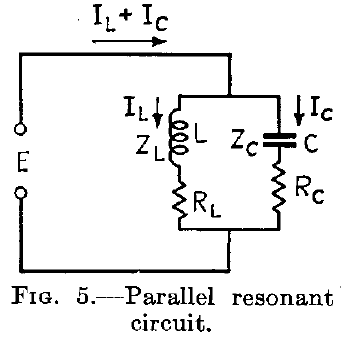# Series RLC Circuits: Resonant Frequency of 60° at 40 Hz

• Butterfly41398

#### Butterfly41398

Poster has been reminded to post schoolwork in the Homework Help forums

A series RLC circuit with R = 250 ohms and L = 0.6 H results in a leading phase angle of 60° at a frequency of 40 Hz. At what frequency will the circuit resonate?

#### Attachments

If "resonance" meaning it is "impedance=0" it is not possible for ever any frequency, but it is a minimum impedance when XL=XC
 Z=[(XL-XC)^2+R^2] and it is for 78.707 Hz

At what frequency will the circuit resonate?
What is your definition for "resonance"?
I ask this because there exist some confusion concerning this term.
I think, the most general definition is: Zero phase between voltage and current - identical to a pure real resistance. In some simple cases this requirement is identical to voltage maximum (bandpass) or minimum (bandstop), but this is nothing else than the RESULT of applying the above definition.

Is this homework?

There are two separate problems here.
1. Identify the value of capacitance that will give a leading phase of 60° at 40 Hz.
2. Identify the frequency at which XL + XC = zero.

Unfortunately I am having trouble reading you work.

... but it is a minimum impedance when XL=XC
Since XC is negative, and XL is positive, resonance is when XL + XC = 0.
At the resonant frequency the total reactance is zero.
The impedance is not zero at resonance, the impedance is purely resistive.

What is your definition for "resonance"?
I ask this because there exist some confusion concerning this term.
I think, the most general definition is: Zero phase between voltage and current - identical to a pure real resistance. In some simple cases this requirement is identical to voltage maximum (bandpass) or minimum (bandstop), but this is nothing else than the RESULT of applying the above definition.
Fort a series RLC circuit, minimum impedance coincides with zero phase difference. For a a parallel RLC circuit, the two do not quite coincide. But this is only noticable when the Q is very low.

Fort a series RLC circuit, minimum impedance coincides with zero phase difference. For a a parallel RLC circuit, the two do not quite coincide. But this is only noticable when the Q is very low.
For ideal RLC components, I find that hard to believe mathematically. Maybe you are considering the more complex situation where the inductor in a parallel RLC circuit also has some series wire resistance?

For ideal RLC components, I find that hard to believe mathematically. Maybe you are considering the more complex situation where the inductor in a parallel RLC circuit also has some series wire resistance?
First of all my apologies for a slip, that the parallel resonant circuit exhibits high not low impedance.
But if you look at Radio Engineering by Terman, or if you draw a phasor diagram, for pure L, C and R in parallel, you will notice that zero phase does not coincide with max impedance. This is only noticeable when the Q is very low, less than say 5. Q may be defined as R/XL for this purpose.

But if you look at Radio Engineering by Terman, or if you draw a phasor diagram, for pure L, C and R in parallel, you will notice that zero phase does not coincide with max impedance. This is only noticeable when the Q is very low, less than say 5. Q may be defined as R/XL for this purpose.
Terman wrote that early in the 1940s and employed at the time a different definition of the RLC circuits.

For the series RLC circuit, the R component was made from the sum of the L and C series resistances.
Termans series circuit was symbolically ( RL + RC ) + jXL + jXC;

For the parallel circuit, the R components remained in series with both the L and C components, before the subcircuits were placed in parallel.
Termans parallel circuit was symbolically ( RL + jXL ) // ( RC + jXC )That explains why Terman wrote; “Furthermore, the details of the behavior of a low Q parallel circuit also depend upon the division of resistance between the inductive and capacitive branches, upon the way in which the resistance varies with frequency, and upon whether the adjustment to resonance is made by varying the frequency, inductance, or capacity.”

The convention now is to assume ideal components with a single R in series or in parallel with ideal L and C components.